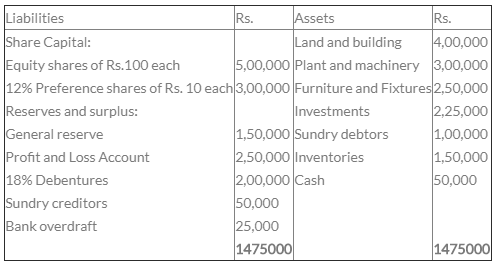# Test: Redemption Of Preference Shares - 2

## 25 Questions MCQ Test Crash Course of Accountancy - Class 12 | Test: Redemption Of Preference Shares - 2

Description
Attempt Test: Redemption Of Preference Shares - 2 | 25 questions in 30 minutes | Mock test for CA Foundation preparation | Free important questions MCQ to study Crash Course of Accountancy - Class 12 for CA Foundation Exam | Download free PDF with solutions
QUESTION: 1

Solution:
QUESTION: 2

Solution:
QUESTION: 3

### X Ltd. had 5,000 12% Redeemable Preference Shares of Rs. 100 each. The company decided to redeem them by issuing equity shares of Rs. 100 each @ a premium of 255. The member of equity shares to be issued are:

Solution:
QUESTION: 4

Which of the following can be utilized for redemption of preference shares?

Solution:
QUESTION: 5

Ajay Ltd. decides to redeem 10,000 Preference Shares of Rs. 10 each at 10% premium. Balance in Profit and Loss A/c is Rs. 65,000 and in Securities Premium A/c is Rs. 5,000. You are required to calculate the minimum number of equity shares of Rs. 10 each to be issued for the purpose of redemption, if the new share is to be issued at a discount of 20%.

Solution:
QUESTION: 6

Preference shares amounting to Rs. 2,00,000 are redeemed at a premium of 5%, by issue of shares amounting to Rs. 1,00,000 at a premium of 10%. The amount to be transferred to capital redemption reserve =?

Solution:
QUESTION: 7

Preference shares cannot be redeemed unless they are:-

Solution:
QUESTION: 8

S Ltd. issued 2,000, 10% Preference shares of Rs. 100 each at par, which are redeemable at a premium of 10%. For the purpose of redemption, the company issued 1,500 Equity Shares of Rs. 100 each at a premium of 20% per share. At the time of redemption of Preference shares, the amount to be transferred by the company to the Capital Redemption Reserve Account =?

Solution:
QUESTION: 9

Which of the following can be utilized for redemption of preference shares?

Solution:
QUESTION: 10

O Ltd. has redeemed its 12% preference shares of Rs. 2,00,000 at a premium of 4%. To meet the redemption it has issued Rs. 1,98,084 worth of shares of Rs. 20 each at a premium of 5%. The balance outstanding to the credit of share premium account after adjusting premium on redemption of preference shares =?

Solution:
QUESTION: 11

Which of the following cannot be used for the purpose of creation of capital redemption reserve account?

Solution:
QUESTION: 12

The Balance sheet of A Ltd. as on March 31,2006 is as under:The 12% preference shares are redeemable at a premium of 10%. The company wishes to maintain the cash balance at Rs. 25,000. For the purpose of redemption of preference shares, it proposed to sell the investments for Rs. 2,00,000. The company proposes to issue sufficient number of equity shares of Rs. 100 each at a premium of 5% to raise required cash resources.
Total Cash required to effect the above decisions is __________.

Solution:
QUESTION: 13

Incase of issue of shares, amount received above per value is credited to which account?

Solution:
QUESTION: 14

Preference shares can be redeemed :

Solution:
QUESTION: 15

Redeemable preference shares must be redeemed within :

Solution:
QUESTION: 16

Which of the following statements is false ?

Solution:
QUESTION: 17

O Ltd. has redeemed its 12% preference shares of Rs. 2,00,000 at a premium of 4%. To meet the redemption it has issued Rs. 1,98,084 worth of shares of Rs. 20 each at a premium of 5%. The balance outstanding to the credit of share premium account after adjusting premium on redemption of preference shares =?

Solution:
QUESTION: 18

During the year 2000-2001, T Ltd. issued 20,000, 12% Preference Shares of Rs. 10 each at a premium of 5%, which are redeemable after 4 years at par. During the year 2005-2006, as the company did not have sufficient cash resources to redeem the preference shares, it issued 10,000,14% debentures of Rs. 10 each at a premium of 10%. At the time of redemption of 12% preference shares, the amount to be transferred to capital redemption reserve =?

Solution:
QUESTION: 19

Redemption of Preference Shares

Solution:
QUESTION: 20

Which of the following statement is true?

Solution:
QUESTION: 21

Securities premium cannot be used to ___________.

Solution:
QUESTION: 22

A preference share which carry the right of participating in the surplus left after paying equity dividend is called:

Solution:
QUESTION: 23

Redeemable Preference shares of Rs. 1,00,000 are redeemed at par for which fresh equity shares of Rs. 80,000 are issued at discount of 10%. The amount transferred to Capital Redemption Reserve will be:

Solution:
QUESTION: 24

Preference Shares can be issued for a maximum period :

Solution:
QUESTION: 25

A preference share which carry the right of participating in the surplus left after paying equity dividend is called:

Solution:Use Code STAYHOME200 and get INR 200 additional OFF Use Coupon Code